# Aquarium

I have an aquarium that is 100 cm long and 40 cm wide and 40 cm in height. We fill it with water. How much will it weigh?

m =  160 kg

### Step-by-step explanation: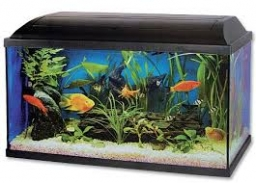Did you find an error or inaccuracy? Feel free to write us. Thank you!Tips to related online calculators
Tip: Our volume units converter will help you with the conversion of volume units.
Tip: Our Density units converter will help you with the conversion of density units.

#### You need to know the following knowledge to solve this word math problem:

We encourage you to watch this tutorial video on this math problem:

## Related math problems and questions:

• Empty aquariumHow much does an empty aquarium weigh with dimensions: length = 40 cm, width = 30 cm, height = 20 cm, if 1 dm2 of glass weighs 300 g? Calculate its weight in kilograms.
• AquariumTry to estimate the weight of the water in an aquarium 50cm long 30cm wide, when poured to a height of 25cm. Calculates the weight of the aquarium's water.
• Aquarium 6How high is the water level in the aquarium with a rectangular base 40cm and 50cm if it is filled 0,65hl of water?
• An aquariumAn aquarium tank that measures 2.4 m high, 6 m long, and 1.5 m wide and is completely filled with water. The density of water is 1,000 kg/m3. What is the mass of the water in the tank?
• Water tank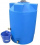The block-shaped tank is 2.5 m long, 100 cm wide and 12 dm high. In how many minutes will it be filled with water to two thirds if 40 liters of water flow into the tank per minute?
• Glass aquarium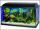How many m2 of glass are needed to produce an aquarium with bottom dimensions of 70 cm x 40 cm and 50 cm high?
• MilimetersThe pool is 6 meters long, 3 meters wide and the water in it is filled with water to a height 1.7 m. When John jumped into it and completely submerged, the level has risen by 5.4 mm. How much weight John when we know that one liter of the human body weigh
• Snails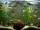How many liters of water will fit in an aquarium with bottom dimensions of 30 cm and 25 cm and a height of 60 cm, if we pour water up to a height of 58 cm? How many most snails can we keep in an aquarium, if we know that snails need 600 cm ^ 3 of water fo
• Aquarium heightHow high does the water in the aquarium reach, if there are 36 liters of water in it? The length of the aquarium is 60 cm and the width is 4 dm.
• AquariumFind how many dm2 of glass we need to make a block-shaped aquarium (the top is not covered) if the dimensions of the aquarium are to be: width 50 cm, length 120 cm, and height 8.5 dm.
• Stone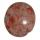When Peter threw stone in a box of water he discovered that the water level has risen by 6 cm. The box has a cuboid shape, the bottom has dimensions of 24 cm and 14 cm, height is 40 cm. What volume has a stone?
• AquariumHow many liters of water can fit in an aquarium measuring 30, 15 and 20 cm?
• The wall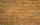We have to build a cuboid wall with dimensions base 30 cm and 45 cm and height 3.25 meters. Calculate how many we need bricks if we spend 400 pieces of bricks to 1 m3 of wall?
• Bathtub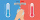Kevin has a bathtub shaped like a block. The bathtub measures 70 cm long, 45 cm wide and 50 cm high. Then the bathtub will be filled with clean water 3/5 of its height. The clean water needed is….
• Swimming pool 4The pool shaped cuboid measuring 12.5 m × 640 cm at the bottom is 960hl water. To what height in meters reaches the water level?
• Concrete boxThe concrete box with walls thick 7 cm has the following external dimensions: length 1.8 m, width 44 cm and height 46 cm. How many liters of soil can fit if I fill it to the brim?
• Perpendicular prism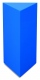Calculate the volume of the perpendicular prism if its height is 60.8 cm and the base is a rectangular triangle with 40.4 cm and 43 cm legs.